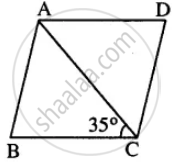# Abcd is a Rhombus. If ∠Bca = 35°. Find ∠Adc. - Mathematics

Sum

ABCD is a rhombus. If ∠BCA = 35°. find ∠ADC.

#### Solution

Given : Rhombus ABCD in which ∠BCA = 35°∠DAC = ∠BCA (Alternate ∠s)

But ∠BCA = 35° (Given)

∠DAC = 35°

But ∠DAC = ∠ACD ( AD = CD) & ∠DAC +∠ACD + ∠ADC = 180°

35°+ 35° + ∠ADC = 180°

∠ADC = 180° – 70° = 110°

Concept: Types of Quadrilaterals - Properties of Rhombus
Is there an error in this question or solution?

#### APPEARS IN

Selina Concise Mathematics Class 8 ICSE
Chapter 17 Special Types of Quadrilaterals
Exercise 17 | Q 7 | Page 198